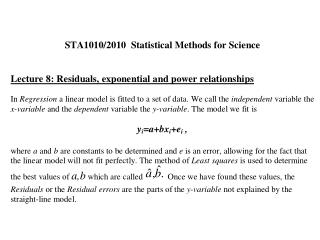# Warning! - PowerPoint PPT PresentationDownload PresentationWarning!

Warning!
Download Presentation## Warning!

- - - - - - - - - - - - - - - - - - - - - - - - - - - E N D - - - - - - - - - - - - - - - - - - - - - - - - - - -
##### Presentation Transcript

1. Warning! Excel 97 (and almost all other statistical software and textbooks) define Residuals the same way as in this course: Residual = Observed value – Fitted Value But Excel 2000 uses Residual = Fitted Value – Observed Value Result: Residual Plots from Excel 2000 are upside down!

2. Logarithms and Exponential Relationships Basic Rules: ln(a x b) = ln (a) + ln(b) ln(ex) = x, Equivalently, e(ln y) = y

3. Some Special Cases: • ln(y) = ln(a) + bx equivalent to y = aebx • Examples: • ln(y) = 3 + 2 x => y = ?? • y = 5e-1.7x => ln(y) = ??

4. Some Special Cases: • ln(y) = ln(a) + bx equivalent to y = aebx • Examples: • ln(y) = 3 + 2 x => y = ?? • y = 20.08e2x • y = 5e-1.7x => ln(y) = ?? • ln(y) = 1.609 – 1.7 x

5. Example 2: Power Law y = axb equivalent to ln(y) = ln(a) + b ln(x) Examples: y = 7x3 => ln(y) = ?? ln(y) = -0.2 + 1.5 ln(x) => y = ??

6. Example 2: Power Law y = axb equivalent to ln(y) = ln(a) + b ln(x) Examples: y = 7x3 => ln(y) = ?? ln(y) = 1.946 + 3 ln(x) ln(y) = -0.2 + 1.5 ln(x) => y = ?? y = 0.819 x1.5